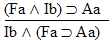Logic Chapter Thirteen
Next Chapter

The Stile

The "," called the "stile" and pronounced "IF'N'ONLYIF," or ("iffen-oh-knee-ef" or "iffen-on-lee-ef") joins two statements together to make a new statement (called a "biconditional") which is true only when those two statements have the same truth value, and false only when they have different truth values. Here's the truth table. Think of it as saying "has the same truth value as" or "is logically equivalent to." (But not as saying "is.") Here's it's truth table.Notice that unlike every other operator, this one has the same number of Fs and Ts.
For practice, work out the values of these biconditionals in these worlds.BaCa BbCa BaBb BbBb CaCb BbBa BaCb BbCb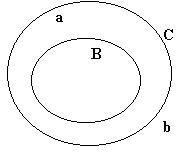BaCa BbCa BaBb BbBb CaCb BbBa BaCb BbCb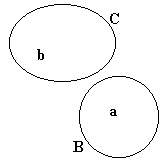BaCa BbCa BaBb BbBb CaCb BbBa BaCb BbCb

To recap, here's a truth table for the five operators we've got so far.Have you ever wondered how the stile is related to the horseshoe? You haven't? Well, pretend that you have. And study the following truth table.Now ask yourself, what is the value of PQ when Q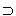P and PQ are both true? For that matter, what are the values of QP and PQ when PQ is true? That's right, this biconditional is true if and only if both of these conditionals are true.

Some of the following arguments are valid, some aren't. You figure it out! (Before you click on each argument for the answer)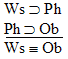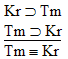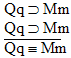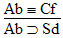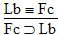If you have time, go through these arguments in two ways. First, just look at them and think about the logical relationships between the formulas. See if you can figure out which arguments are valid and which aren't just by working through the formulas in your own mind. Then, when you've given each one a good try, do a truth table for each argument, or at least for the most difficult arguments.

When you've done at least one truth table for a valid argument, and at least one truth table for an invalid argument, you can go on to the next section. Don't go on until you've done at least some practice work.

Rules for biconditionals

Remember that the value of PQ is only true when QP and PQ are both true, and that are the values of QP and PQ are both true when PQ is true. In other words, this biconditional is true if and only if both of these conditionals are true. Which suggests a couple of rules.

 Rule 14: Pack Biconditional (PB)  If QP and PQ are both available lines, then PQ may be written as a new line in the derivation.

 Rule 15: Unpack Biconditional (UB)  If PQ is an available line, then QP or PQ may be written as a new line in the derivation.

Here's these two rules in action.is a valid argument, and here's the proof: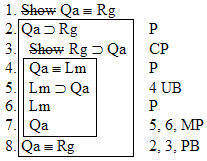Here's a whole bunch of arguments to practice on. Prove the invalid ones invalid by truth table, or by writing out a truth table row that proves the argument invalid. Prove the valid ones's valid by derivation. If you're not sure whether a particular argument is valid, figure it out by the truth table method.
 1.2.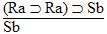3.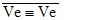4.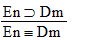5.6.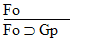7.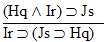8.9.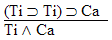10.11.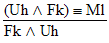12.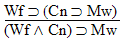13.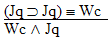14.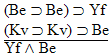15.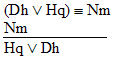16.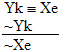17.18.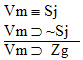19.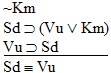20.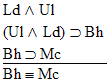21.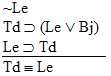22.23.24.25.26.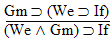27.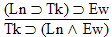28.